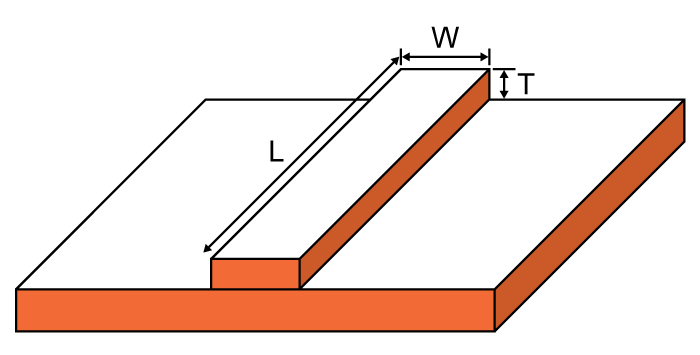# Trace Resistance Calculator

## This calculator determines the resistance of a microstrip trace

Ohms

### Overview

The trace resistance of a microstrip is important in determining how much power it dissipates. By determining its trace resistance, the contribution of a microstrip to the overall circuit resistance can be evaluated. This tool is designed to calculate the resistance of a microstrip trace with a copper conductor. To use it, specify the trace dimensions and the ambient temperature.### Equation

$$R=\rho \cdot \frac{L}{T\cdot W}\cdot \left [ 1+\alpha\cdot \left ( temp-25 \right ) \right ]$$

Where:

ρ=resistivity

L= length

W=trace width

T=trace height

Note:

ρcopper  =  1.7 x 10-6 ohm-cm
αcopper (temperature coefficient) = 3.9 x 10-3 ohm/ohm/C

### Applications

The microstrip is a popular device in microwave radio technology. It was invented because of the physical limitations to the manufacturing of inductors and capacitors at such very high frequencies. A microstrip is made out of printed circuit boards whose dimensions are carefully set to meet required parameters. One of those required parameters is the trace resistance.

From Ohm's law, we know that resistance is inversely proportional to current. That is, the lower the resistance, the higher the current and vice versa. Current is also proportional to power according to P = VI. Thus, resistance also becomes a factor when calculating power consumption. It is important to determine the resistance of the trace of a microstrip so that the power dissipated by it can be determined.• A
albertopyhy9 October 15, 2021

A microstrip is made out of printed circuit boards whose dimensions are carefully set to meet required parameters. | El Dorado Hills Therapist

Like.
•mariaalmendrada October 21, 2021

In many PCB trace width calculators, I’ve noticed the resistance of the trace will depend on its ubication. In other words, the resistance is not the same in the inner and external layer. However, this is not taken into account in this formula. Any idea why the resistance change then?

Like.
• D
dyrkane1 January 18, 2022
The resistance depends on ubication because internal traces have lower thermal impedance compared to external traces. So internal traces run cooler and resistance is lower.
Like.